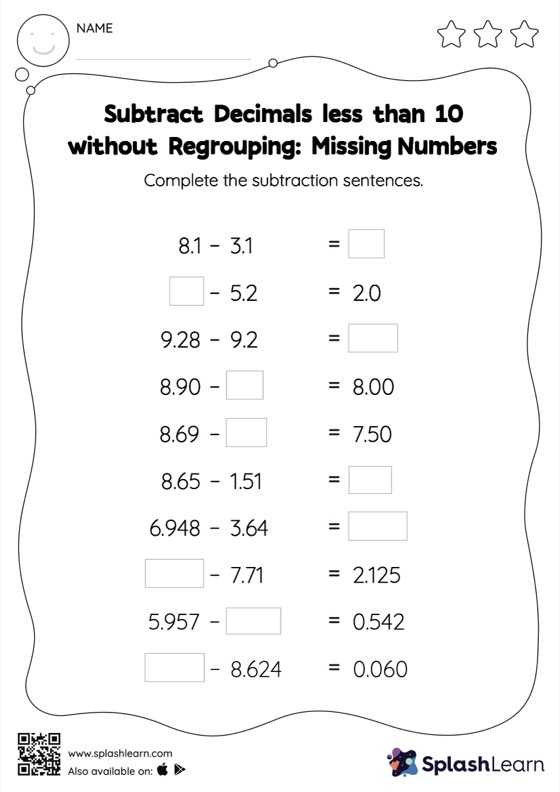# Subtract Decimals less than 10 without Regrouping: Missing Numbers Worksheet

Home > Subtract Decimals less than 10 without Regrouping: Missing NumbersThis worksheet challenges young mathematicians to hone their math skills by solving a set of problems on subtracting decimals less than 10 without regrouping. Students use the relationship between addition and subtraction to find the missing number while subtracting decimals. They do not need to regroup the numbers in subtract decimals less than 10 without regrouping worksheet to get to the result. In each problem, the numbers are laid out in the horizontal format. Students should try to use different strategies involving composing and decomposing numbers to solve these problems. This will help them develop flexibility and fluency.